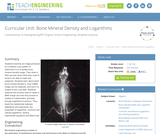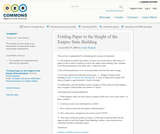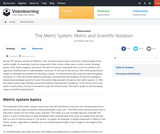# Search Results (5)

View
Selected filters:
• ExponentConditions of Use:
Rating

Students examine an image produced by a cabinet x-ray system to determine if it is a quality bone mineral density image. They write in their journals about what they need to know to be able to make this judgment. Students learn about what bone mineral density is, how a BMD image can be obtained, and how it is related to the x-ray field. Students examine the process used to obtain a BMD image and how this process is related to mathematics, primarily through logarithmic functions. They study the relationship between logarithms and exponents, the properties of logarithms, common and natural logarithms, solving exponential equations and Beer's law.

Subject:
Engineering
Life Science
Physics
Material Type:
Unit of Study
Provider:
TeachEngineering
Provider Set:
TeachEngineering
Author:
Kristyn Shaffer
09/18/2014Conditions of Use:
No Strings Attached
Rating

According to the GED testing service, test takers struggle with “applying rules of exponents in numerical expressions with rational exponents to write equivalent expressions with rational exponents.” (https://www.gedtestingservice.com/uploads/files/09738c12fe4e4accd9a16bab7cb99a3c.pdf )
Students do “fairly well” with simple squares and square roots, but there is a “sharp drop-off” when things get more complicated.
These are questions included in the “no calculator” portion of the test.
These skills are Mathematics Standards Level D in the College and Career Readiness Standards for Adult Education (https://www.educateiowa.gov/sites/files/ed/documents/CCRStandardsAdultEd.pdf ) under “Expressions and Equations.”
This curriculum guide will offer opportunities to build the deeper understanding necessary to understand the rules of exponents such as (xm)n = xmn .
Write and evaluate numerical expressions involving whole-number exponents. (6.EE.1)
Know and apply the properties of integer exponents to generate equivalent numerical expressions. (8.EE.1)

Subject:
Mathematics
Material Type:
Activity/Lab
Lesson Plan
Teaching/Learning Strategy
Author:
Susan Jones
05/25/2018Conditions of Use:
Remix and Share
Rating

This activity can be used as an introduction to exponents and/or exponential expressions.

Subject:
Mathematics
Material Type:
Activity/Lab
Author:
Cindy Shepard
01/28/2016Rating

This lesson describes the history and basic operation of the metric system as well as scientific notation. The simplicity of the metric system stems from the fact that there is only one unit of measurement (or base unit) for each type of quantity measured (length, weight, etc.).

Subject:
Education
Mathematics
Astronomy
Material Type:
Interactive
Unit of Study
Provider:
UCAR Staff
Provider Set:
Visionlearning
Author:
Anthony Carpi
01/01/2000Conditions of Use:
Remix and Share
Rating

In this seminar you will explore square roots and see how they are used throughout mathematics.  You will see how “squaring” a number and “taking the square root” relate to each other. Also presented will be how to estimate square roots when given a number. You will have plenty of opportunity to try practice problems, review, and even create a short presentation. You will apply techniques you have learned involving estimating. You will use the techniques learned in this seminar to solve problems involving square roots moving forward and ultimately be able to verify solutions. When working with square roots, you will identify the patterns in perfect square numbers, as well as in techniques such as squaring and cubing numbers.  By the end, you should have a better understanding of what a square root is and how to handle expressions that involve square roots.StandardsCC.2.1.HS.F.1Apply and extend the properties of exponents to solve problems with rational exponents.CC.2.2.HS.D.6Extend the knowledge of rational functions to rewrite in equivalent forms.

Subject:
Mathematics
Algebra
Material Type:
Lesson Plan
Author:
Tracy Rains